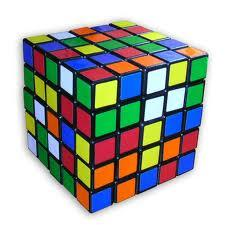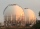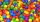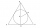# Transforming cuboid

Cuboid with dimensions 6 cm, 10, and 11 cm is converted into a cube with the same volume. What is its edge length?

Correct result:

x =  8.7 cm

#### Solution:

$a=6 \ \text{cm} \ \\ b=10 \ \text{cm} \ \\ c=11 \ \text{cm} \ \\ \ \\ V=a \cdot \ b \cdot \ c=6 \cdot \ 10 \cdot \ 11=660 \ \text{cm}^3 \ \\ \ \\ V=x^3 \ \\ \ \\ \ \\ x=\sqrt{ V}=\sqrt{ 660 }=8.7 \ \text{cm}$Our examples were largely sent or created by pupils and students themselves. Therefore, we would be pleased if you could send us any errors you found, spelling mistakes, or rephasing the example. Thank you!

Please write to us with your comment on the math problem or ask something. Thank you for helping each other - students, teachers, parents, and problem authors.Tips to related online calculators
Looking for a statistical calculator?
Do you want to convert length units?
Tip: Our volume units converter will help you with the conversion of volume units.

## Next similar math problems:

• Four prismsQuestion No. 1: The prism has the dimensions a = 2.5 cm, b = 100 mm, c = 12 cm. What is its volume? a) 3000 cm2 b) 300 cm2 c) 3000 cm3 d) 300 cm3 Question No.2: The base of the prism is a rhombus with a side length of 30 cm and a height of 27 cm. The heig
• Flower boxesHow many m2 of 10mm thick boards are needed to make 12 flower boxes? The dimensions of the box are 180,150 and 1500 mm.
• Aquarium heightHow high does the water in the aquarium reach, if there are 36 liters of water in it? The length of the aquarium is 60 cm and the width is 4 dm.
• Truncated cone 6Calculate the volume of the truncated cone whose bases consist of an inscribed circle and a circle circumscribed to the opposite sides of the cube with the edge length a=1.Calculate the volume of a quadrilateral pyramid, the base of which has the shape of a rectangle with dimensions a = 6cm, b = 4cm and height v = 11cm
• Cylinder containerThe cylindrical container with a diameter of 1.8 m contains 2,000 liters of water. How high does the water reach?
• Gas sphereThe gas tank has the shape of a sphere with a diameter of 14 m. How many m3 of gas will fit in it?
• Clay ballsHow many clay balls with a radius of 1 cm can be made from a ball of clay with a radius of 8 cm?
• Cube surfce2volumeCalculate the volume of the cube if its surface is 150 cm2.
• Volume ratioCalculate the volume ratio of balls circumscribed (diameter r) and inscribed (diameter ϱ) into an equilateral rotating cone.
• Equilateral coneWe pour so much water into a container that has the shape of an equilateral cone, the base of which has a radius r = 6 cm, that one-third of the volume of the cone is filled. How high will the water reach if we turn the cone upside down?
• Concrete hatchThe concrete hatch for a round well has a diameter of 1300 mm and a thickness of 80 mm. Determine its weight in kg if the density of the concrete is 2545 kg/m3
• Hexagonal pyramidFind the volume of a regular hexagonal pyramid, the base edge of which is 12 cm long and the side edge 20 cm.
• Shell area cyThe cylinder has a shell content of 300 cm square, while the height of the cylinder is 12 cm. Calculate the volume of this cylinder.
• The cylinderThe cylinder has a surface area of 300 square meters, while the height of the cylinder is 12 m. Calculate the volume of this cylinder.
• Spherical segmentCalculate the volume of a spherical segment 18 cm high. The diameter of the lower base is 80 cm, the upper base 60 cm.
• Regular hexagonal prismCalculate the volume of a regular hexagonal prism whose body diagonals are 24cm and 25cm long.